Markets
Futures
Earn
More
Cancel
FAQAccount FunctionsTutorialBinance Fan TokenBinance EarnCrypto Deposit/Withdrawal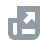Crypto Derivatives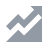FinanceAPISecurityOther Topics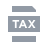Tax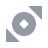Binance Convert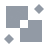NFTVIP
Home
Support Center
FAQ
Tutorial
BNB Balance Calculation

# BNB Balance Calculation

2021-04-19 06:18
The daily BNB balance calculation method has been updated from being recorded in a snapshot at 00:00 (UTC) each day to the Daily Average BNB Balance, which includes BNB held in normal Binance Accounts, Trading Bots Accounts, Sub-Accounts, Margin Accounts, Binance Crypto Loans Collateral, Futures Wallet Accounts, Binance Funding Wallet Accounts, Binance Simple Earn Accounts, BNB Vault Accounts, Launchpool Accounts, DeFi Staking Accounts, Dual Investment Accounts, and Liquidity Farming Accounts.
Total Daily Average BNB Balance = Sum of the daily averages of the following:
• Spot Account Balance
• Sub-Account Balance
• Margin Account Balance
• Binance Crypto Loans Collateral Balance
• Futures Wallet Balance
• Binance Funding Wallet Balance
• Simple Earn Account Balance
• BNB Vault Account Balance
• Launchpool Account Balance
• DeFi Staking Account Balance
• Dual Investment Account Sell High Subscriptions
• Liquidity Farming Account Balance
Note:
• Daily Average Spot Account Balance is calculated using the sum of the hourly snapshots of BNB balance in the spot account in a day divided by 24 (hours).
• Daily Average Trading Bots Account Balance is calculated as the sum of the hourly snapshots of the BNB balance in the Trading Bots Account in a day divided by 24 (hours).
• Daily Average Sub-Account Balance is calculated as the sum of the hourly snapshots of the BNB balance in the sub-account in a day divided by 24 (hours).
• Daily Average Margin Account Balance is calculated as the sum of the hourly snapshots of the net BNB balance (Net BNB Balance = BNB Total Balance - Borrowed BNB - BNB Interest) in both master and sub-account margin accounts in a day divided by 24 (hours). Please note that margin account balances may be a negative balance.
• Daily Average Binance Crypto Loans Account BNB Collateral Balance is calculated as the sum of the hourly snapshots of the BNB collateral balance in the Binance Crypto Loans account in a day divided by 24 (hours).
• Daily Average Binance Futures Wallet Balance is calculated as the sum of the hourly snapshots of the BNB balance in the Binance Futures Account in a day divided by 24 (hours).
• Daily Average Binance Funding Wallet Balance is calculated as the sum of the hourly snapshots of the BNB balance in the Binance Funding Wallet Account in a day divided by 24 (hours).
• Daily Average Simple Earn Account Balance is calculated as the sum of the hourly snapshots of the BNB balance in the Binance Simple Earn Account in a day divided by 24 (hours).
• Daily Average BNB Vault Account Balance is calculated as the sum of the hourly snapshots of the BNB balance in the BNB Vault Account in a day divided by 24 (hours).
• Daily Average Binance Launchpool Account Balance is calculated as the sum of the hourly snapshots of the BNB balance in the Binance Launchpool Account in a day divided by 24 (hours).
• Daily Average DeFi Staking Account Balance is calculated as the sum of the hourly snapshots of the BNB balance in the DeFi Staking Account in a day divided by 24 (hours).
• Daily Average Dual Investment Balance is calculated as the sum of the hourly snapshots of the BNB balance in the Dual Investment Account in a day divided by 24 (hours).
• Daily Average Liquidity Farming Balance is calculated as the sum of the hourly snapshots of the BNB balance in the Liquidity Farming Account in a day divided by 24 (hours).
This update to the BNB balance calculation mechanism will impact the following aspects of the Binance Ecosystem: